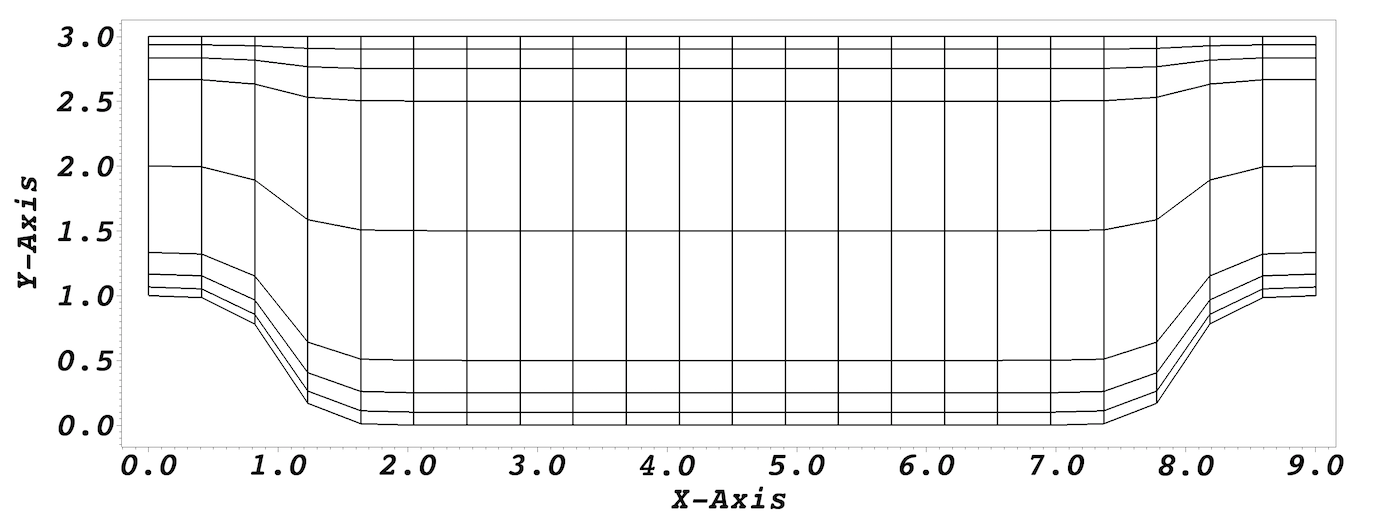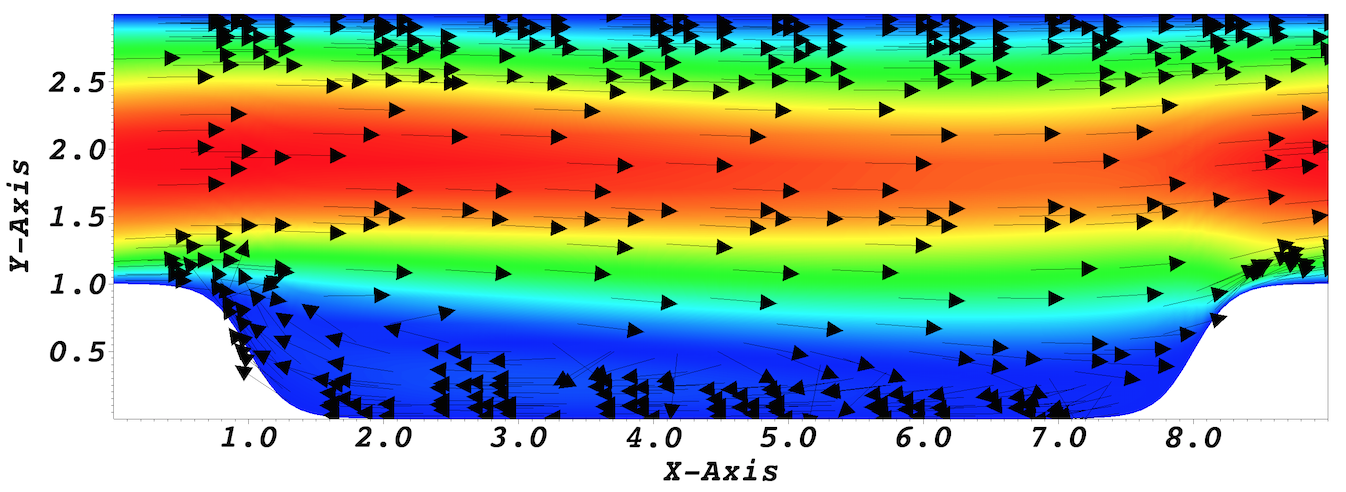# Periodic Hill¶

This tutorial will describe how to run a case from scratch. We illustrate this procedure through a relatively simple example involving incompressible laminar flow in a two-dimensional periodic hill domain. Our implementation is loosely based on the case presented by Mellen et al. [Mellen2000]. A thorough review for this case can be found in the ERCOFTAC knowledge base wiki.

## Pre-processing¶

We assume that you have installed Nek5000 in your home directory. This tutorial requires that you have the tools genbox and genmap compiled. Make sure $HOME/Nek5000/bin is in your search PATH. Cases are setup in Nek5000 by editing case files. Users should select an editor of choice with which to do this (e.g vi). A case being simulated involves data for mesh, parameters, etc. As a first step, the user should create a case directory in their run directory. cd$HOME/Nek5000/run
mkdir hillp
cd hillp


## Mesh generation¶

In this tutorial we use a simple box mesh generated by genbox with the following input file:

-2                     spatial dimension (will create box.re2)
1                      number of fields
#
#    comments: two dimensional periodic hill
#
#========================================================
#
Box                                       hillp
-22 8                                     Nelx  Nely
0.0 9.0 1.                                x0 x1 ratio
0.0 0.1 0.25 0.5 1.5 2.5 2.75 2.9 3.0     y0 y1 ratio
P  ,P  ,W  ,W                             BC's:  (cbx0, cbx1, cby0, cby1)


For this mesh we are specifying 22 uniform elements in the stream-wise (x) direction. 8 non-uniform elements are specified in the span-wise (y) direction in order to resolve the boundary layers. The boundary conditions are periodic in the x-direction and no-slip in the y. Additional details on generating meshes using genbox can be found here. Now we can run genbox with

genbox


On input provide the input file name (e.g. hillp.box). The tool will produce a binary mesh and boundary data file box.re2 which should be renamed to hillp.re2.

## usr file¶

The user file implements various subroutines to allow the user to interact with the solver.

To get started we copy the template to our case directory

cp $HOME/Nek5000/core/zero.usr hillp.usr  ### Modify mesh and apply mass flux¶ To drive the flow a mass flux is applied such that bulk velocity $$u_b=1$$. For a periodic hill, we will need to modify the geometry. Let $${\bf x} := (x,y)$$ denote the old geometry, and $${\bf x}' := (x',y')$$ denote the new geometry. For a domain with $$y\in [0,3]$$ and $$x\in [0,9]$$ the following function will map the straight pipe geometry to a periodic hill: $y'(x,y) = y + C(3-y)\Big\{1+\tanh\big[B(|x-A|-B)\big]\Big\} .$ where $$A=4.5, B=3.5, C=1/6$$. We have chosen these constants so that the height of the hill (our reference length), $$h=1$$. Note that, as $$y \longrightarrow 3$$, the perturbation, goes to zero. So that near $$y = 3$$, the mesh recovers its original form. In Nek5000, we can specify this through usrdat2 in the usr file as follows subroutine usrdat2() implicit none include 'SIZE' include 'TOTAL' integer ntot,i real sa,sb,sc,xx,argx,A1 ntot = lx1*ly1*lz1*nelt sa = 4.5 sb = 3.5 sc = 1./6 do i=1,ntot xx = xm1(i,1,1,1) argx = sb*(abs(xx-sa)-sb) A1 = sc + sc*tanh(argx) ym1(i,1,1,1) = ym1(i,1,1,1) + (3-ym1(i,1,1,1))*A1 enddo ! apply mass flux to drive the flow such that Ubar = 1 param(54) = -1 ! x-direction param(55) = 1.0 ! Ubar return endFig. 6 Modified box mesh graded ### Initial & boundary conditions¶ The next step is to specify the initial conditions. This can be done in the subroutine useric as follows: subroutine useric(ix,iy,iz,ieg) implicit none include 'SIZE' include 'TOTAL' include 'NEKUSE' integer ix,iy,iz,ieg ux = 1.0 uy = 0.0 uz = 0.0 temp = 0.0 return end  For walls and periodic boundaries, nothing needs to be specified in the user file, so userbc can remain unmodified. ## Control parameters¶ The control parameters for any case are given in the .par file. For this case, using any text editor, create a new file called hillp.par and type in the following # # nek parameter file # [GENERAL] stopAt = endTime endTime = 200 variableDT = yes targetCFL = 0.4 timeStepper = bdf2 writeControl = runTime writeInterval = 20 [PROBLEMTYPE] equation = incompNS [PRESSURE] residualTol = 1e-5 residualProj = yes [VELOCITY] residualTol = 1e-8 density = 1 viscosity = -100  In choosing viscosity = -100 we are actually setting the Reynolds number. This assumes that $$\rho \times u_b \times h = 1$$ where $$u_b$$ denotes the bulk velocity and $$h$$ the hill height. We have set the calculation to stop at the physical time of $$T=200$$ (endTime=200) which is roughly 22 flow-thru time units (based on the bulk velocity $$u_b$$ and length of periodic pitch, $$L=9$$). Additional details on the names of keys in the .par file can be found here. ## SIZE file¶ The static memory layout of Nek5000 requires the user to set some solver parameters through a so called SIZE file. Typically it’s a good idea to start from our template. Copy the SIZE.template file from the core directory and rename it SIZE in the working directory: cp$HOME/Nek5000/core/SIZE.template SIZE


Then, adjust the following parameters in the BASIC section

...

! BASIC
parameter (ldim=2)
parameter (lx1=8)
parameter (lxd=12)
parameter (lx2=lx1)

parameter (lelg=22*8)
parameter (lpmin=1)
parameter (lpmax=4)
parameter (ldimt=1)

...


For this tutorial we have set our polynomial order to be $$N=7$$ - this is defined in the SIZE file above as lx1=8 which indices that there are 8 points in each spatial dimension of every element. Additional details on the parameters in the SIZE file are given here.

## Compilation¶

With the hillp.usr, and SIZE files created, we are now ready to compile:

makenek hillp


If all works properly, upon compilation the executable nek5000 will be generated.

## Running the case¶

First we need to run our domain paritioning tool

genmap


On input specify hillp as your casename and press enter to use the default tolerance. This step will produce hillp.ma2 which needs to be generated only once.

Now you are all set, just run

nekbmpi hillp 4


to launch an MPI jobs on your local machine using 4 ranks. The output will be redirected to logfile.

## Post-processing the results¶

Once execution is completed your directory should now contain multiple checkpoint files that look like this:

hillp.f00001
hillp.f00002
...


The preferred mode for data visualization and analysis with Nek5000 is to use Visit/Paraview. One can use the script visnek, to be found in /scripts. It is sufficent to run:

visnek hillp


(or the name of your session) to obatain a file named hillp.nek5000 which can be recognized in Visit/Paraview.

In the viewing window one can visualize the flow-field as depicted in Fig. 7.Fig. 7 Steady-State flow field visualized in Visit/Paraview. Vectors represent velocity. Colors represent velocity magnitude. Note, velocity vectors are equal size and not scaled by magnitude.